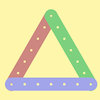#### You may also like### I'm Eight

Find a great variety of ways of asking questions which make 8.### Let's Investigate Triangles

Vincent and Tara are making triangles with the class construction set. They have a pile of strips of different lengths. How many different triangles can they make?### Noah

Noah saw 12 legs walk by into the Ark. How many creatures did he see?

# Secret Number

##### Age 5 to 7Challenge Level
We had a few solutions sent in, here is the one from Samiya from Boxgrove Primary School

because 10 take away 4 equals 6.
Or we can say 4 plus 6 equals 10

Kaiand and Jase from Carrillo Elementary in America wrote;

Her secret number is six. I know this because she never pressed the "=" sign. She only put the + and the 4 and it showed the four. Then when he pressed the "=" sign, it became 10 because 6+4=10.

Abhishek, Xylia,Christopher, Alex, Grace, Seby and Connor from Pakuranga Heights Primary School in New Zealandsent in these two calculations.

4+6=10      5x7=35

Holly from Hermitage School sent a particularly well-explained one:

You know that the number on the addition question is 6 because of two things.
1. I know that 6 + 4 = 10, and 4 was the choosen number, so 6 is what you add on to make 10.
2. You can subtract 4 from 10 and see what the number is. That number is then the secret number, 10 - 4 = 6.

You know that the number on the multiplication question is 7 because of 2 things.
1. I know my 5 times tables and I went through them, {5x1=5,2x5=10,3x5=15,4x5=20,5x5=25,6x5=30,7x5=35}. When I got to 35 I figured out what you times it by.
2. Another way to do it is to do division. 35 divided by 5 is 7. Also if you find division hard you can do 5 jars and 35 sweets.

I think Holly means that you can think of division as sharing, for example sharing out 35 sweets between 5 jars.
Benjy from St Mary Redcliffe Primary looked at the first addition game more generally. He said:

If I ask to add one to the secret number then I can get the number from the answer by taking away one.
If I ask to add one hunded to the secret number then I can get the secret number from the answer by taking away one hundred.

Well done, Benjy - you have got the idea. Charoo from Manor Farm Junior School summed it up by saying:

You know the operation and you know what other number they're using so you do the inverse!

That is indeed one way of doing it, as Holly has shown. Thank you again to all of you who contacted us.